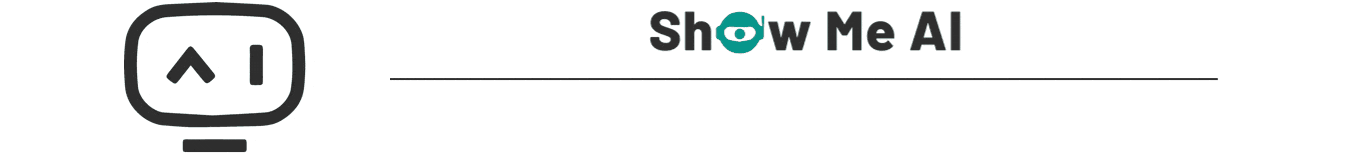# 深度学习与CV教程(11) | 循环神经网络及视觉应用

2022-06-05 20:14 3811 查看### 本篇重点

• RNN的概念与多种形式
• 语言模型
• 图像标注、视觉问答、注意力模型
• RNN梯度流

## 1.RNN的概念与多种形式

### 1.1 形式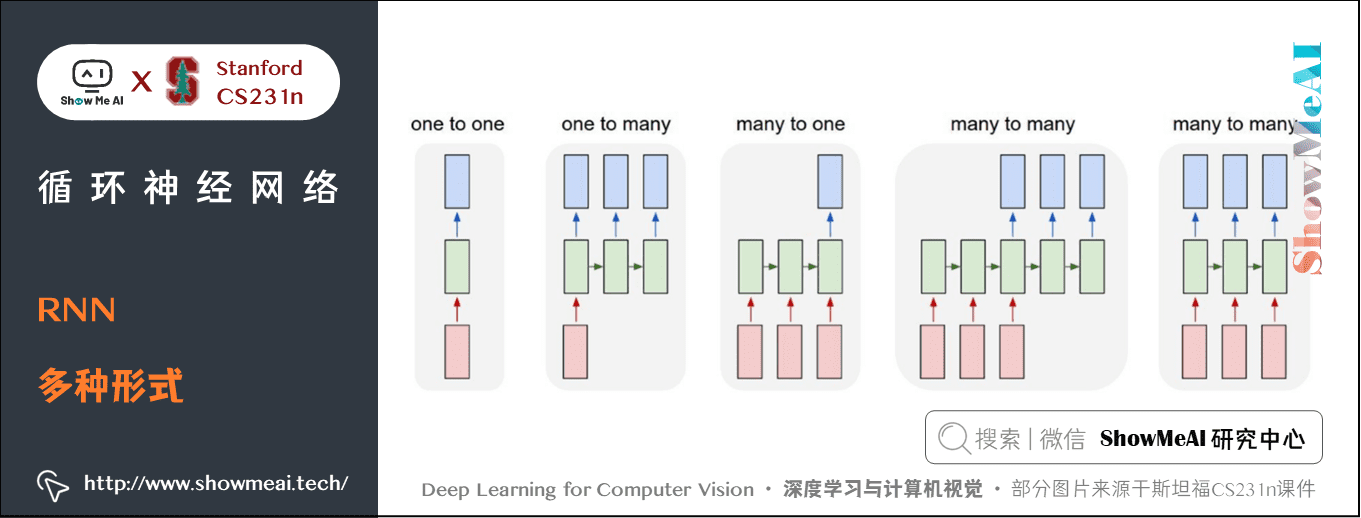#### 5) 多对多（一一对应）

• 比如1张写满数字的固定尺寸的图片，想要识别上面的数字，可以是经过一系列的观察，观察图片的不同部分，然后做出决策；
• 再比如生成一张固定尺寸的图片，上面写满了数字，也可以经过一系列的步骤，一次只能生成一部分。

### 1.2 概念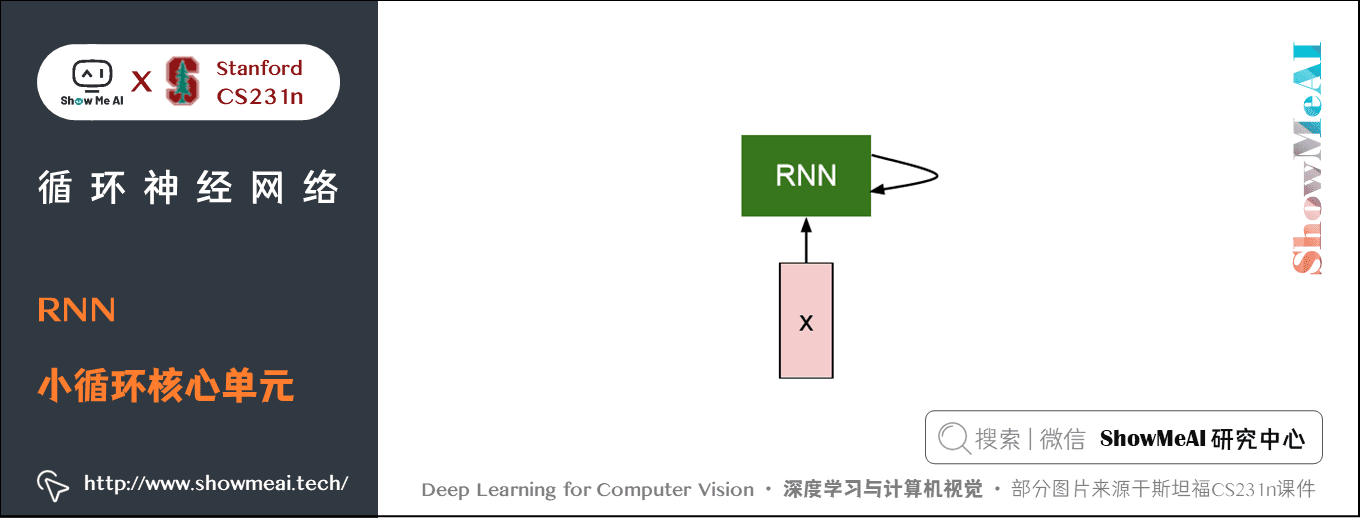• 读取输入 → 更新隐状态 → 基于隐状态计算得到输出。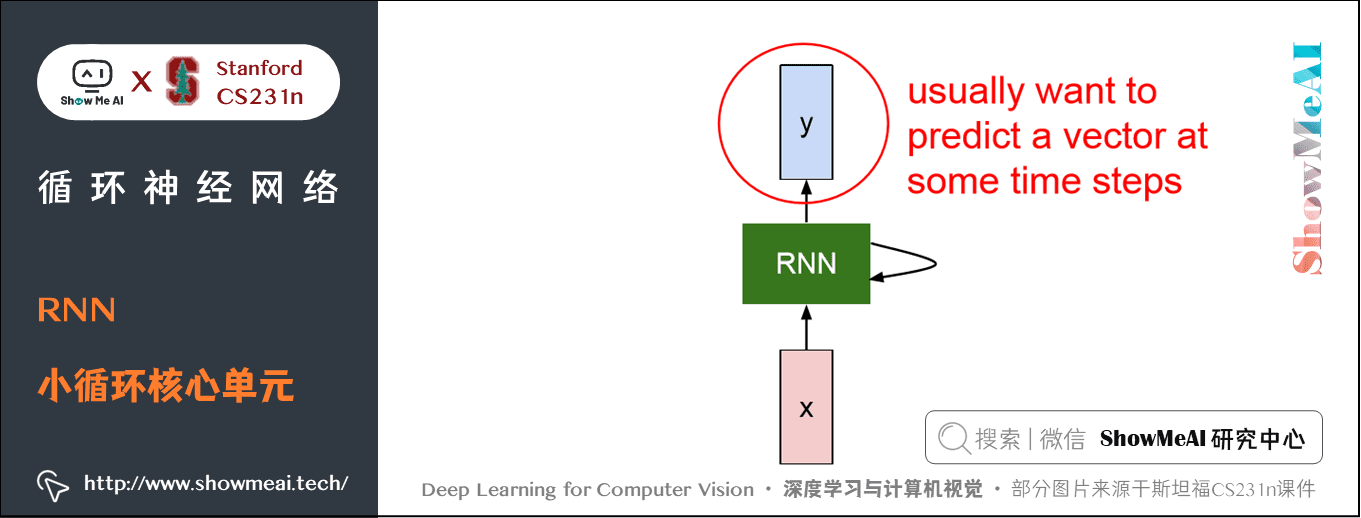h_t=f_W(h_{t-1}, x_t

h_t=tanh(W_{hh}h_{t-1}, W_{xh}x_t) y_t=W_{hy}h_t

### 1.3 计算图

#### 1) 多对多(xy一一对应)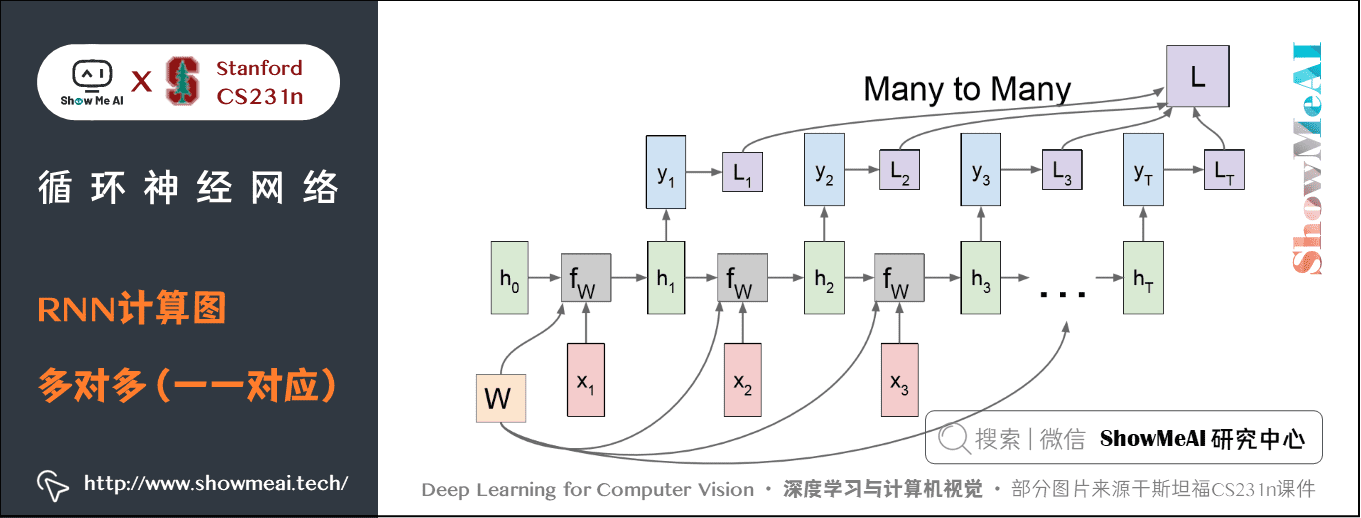#### 2) 多对一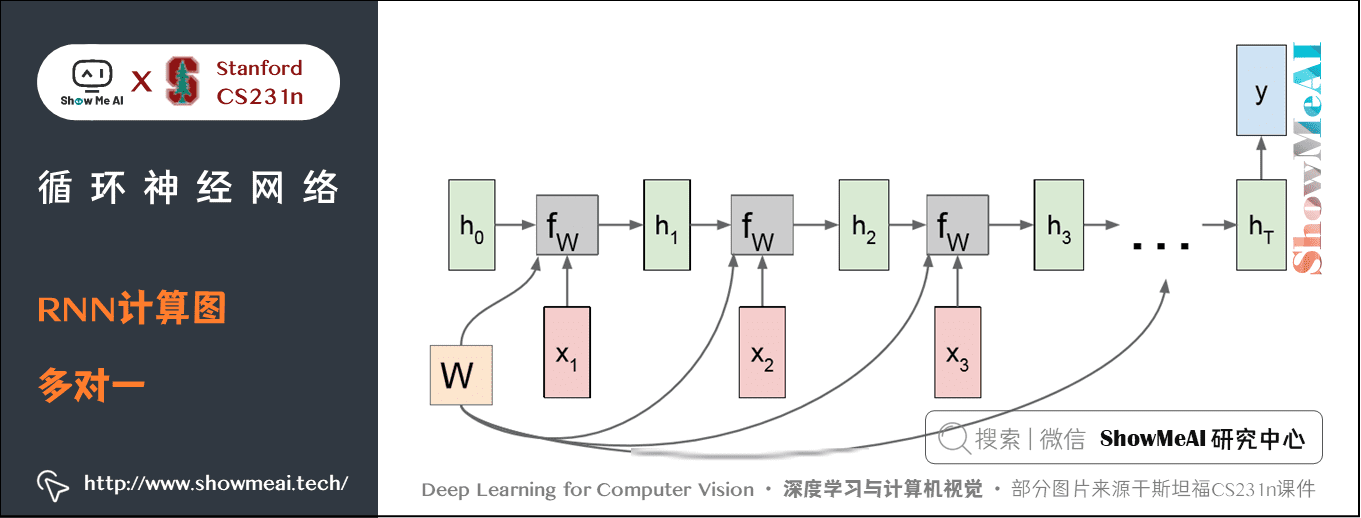#### 3) 一对多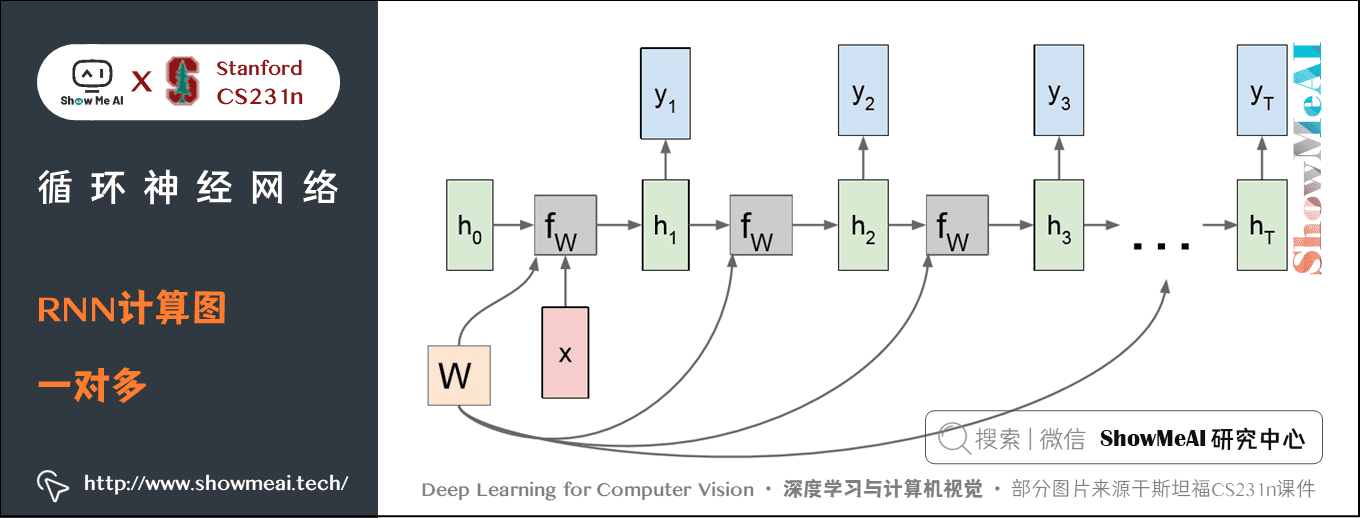#### 4) 多对多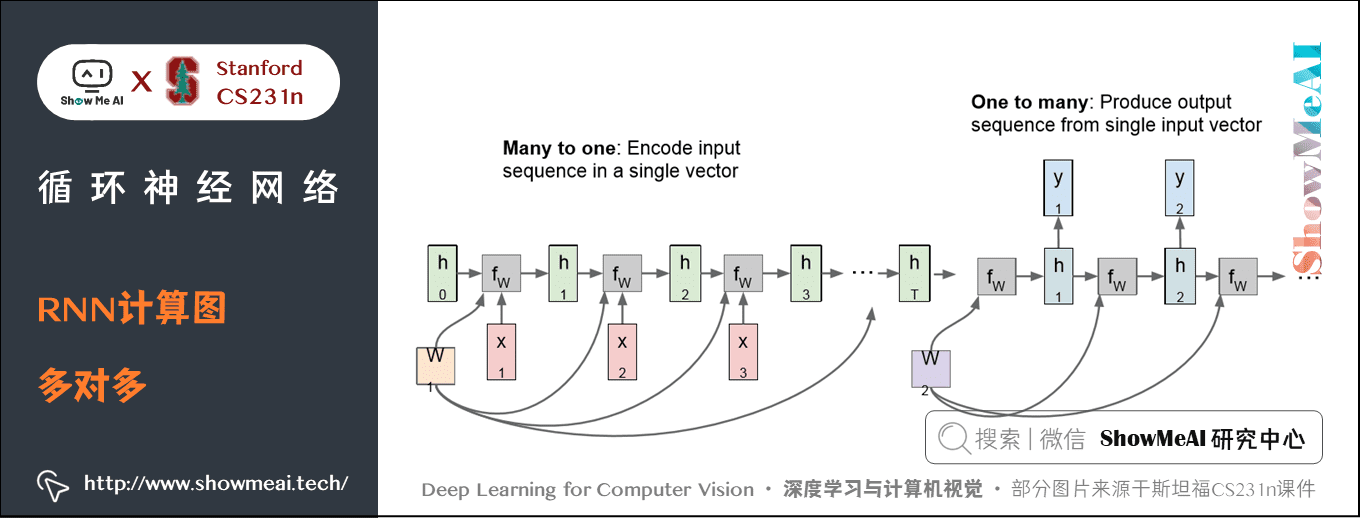## 2.语言模型

[h, e, l, o]

hello
，使用循环公式：

h_t=tanh(W_{hh}h_{t-1}, W_{xh}x_t)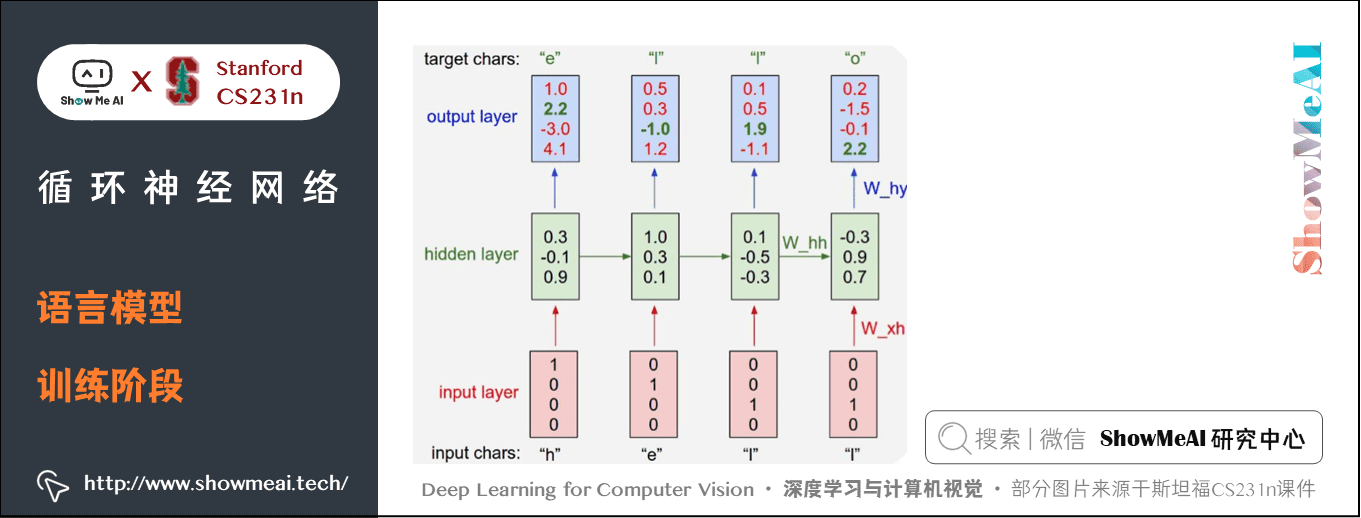h

l

h
，进入第一个RNN单元，然后得到输出 y_1，作为对接下来的字符的一个预测，也就是网络认为的接下来应该输入的字符。

h
，所以接下来输入的应该是
e
，但是模型只是在做预测，如下图所示，模型可能认为接下来要输入的是
o
。在这种错误预测下，我们可以基于 softmax 计算损失来度量我们对预测结果的不满意程度。

e
，利用这个输入和之前的隐状态计算出新的隐状态，然后利用新的隐状态对接下来的字符进行预测，我们希望下一个字符是
l
，但这里模型可能也预测错了，这里又可以计算损失。这样经过不断的训练，模型就会学会如何根据当前的输入预测接下来的输入。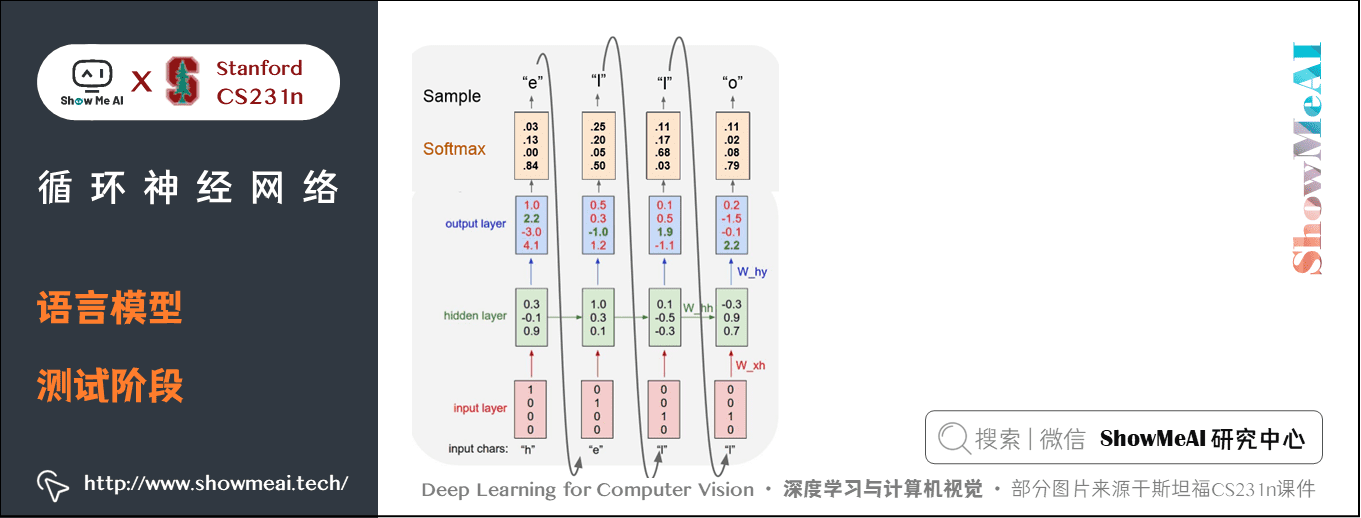h
，现在在RNN的第一步输入
h
，它会产生基于词库所有字母得分的一个 softmax 概率分布，然后使用这个概率分布预测接下来的输出（这个过程叫做sample/采样），如果我们足够幸运得到了字符
e
，然后把这个得到的
e

### 2.1 截断反向传播（Truncated Backpropagation ）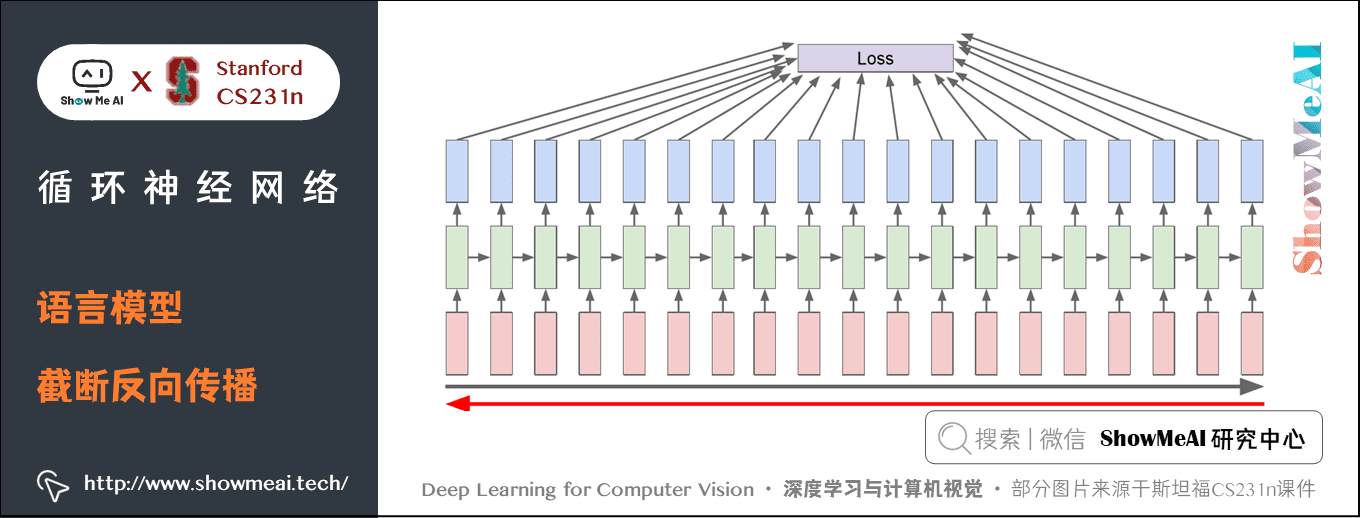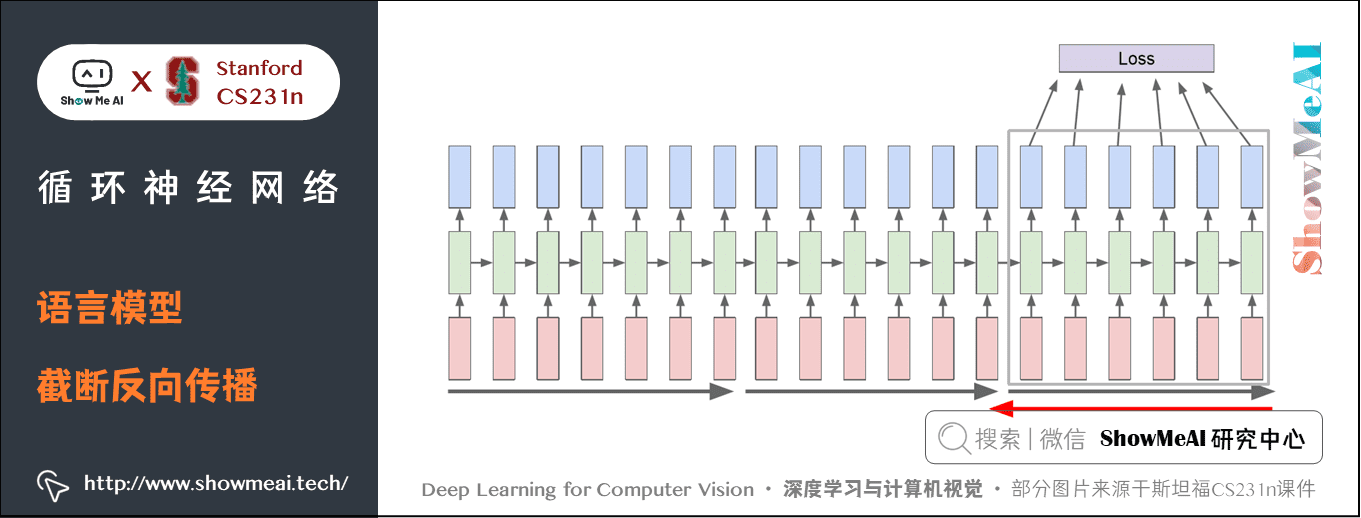### 2.2 RNN语言模型应用

• 使用莎士比亚的文集对语言模型进行训练，然后生成新的文本。结果可以生成莎士比亚风格的文章(当然，结果不是完美的，其中有些部分有错误），如下图所示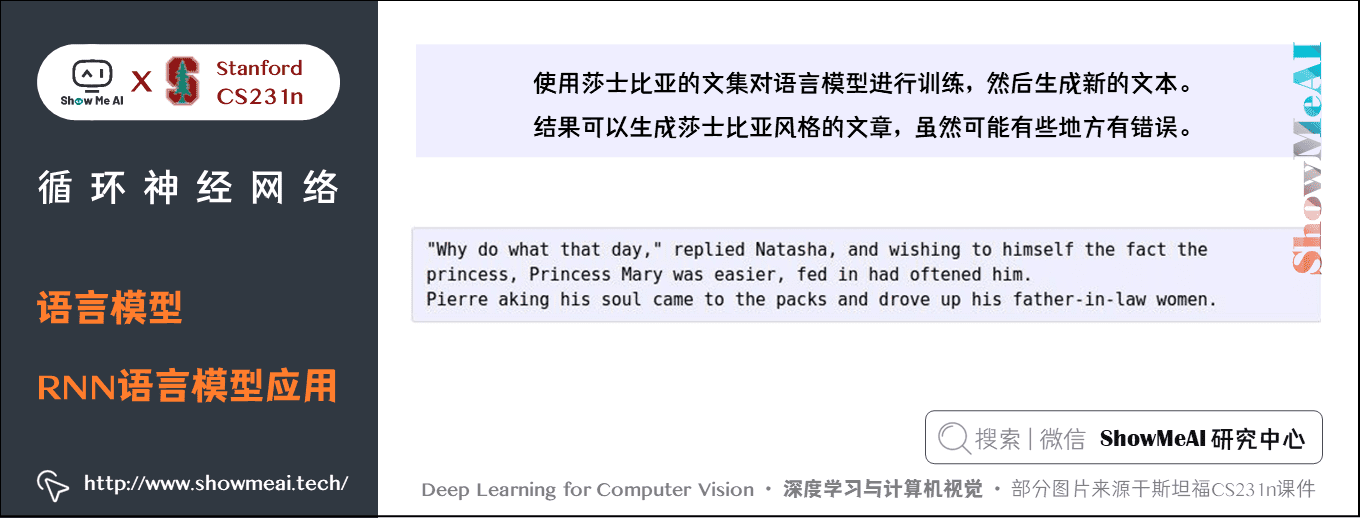• 使用拓扑学教材，可以自动生成一些定理、公式甚至可以画图，虽然都没有意义，但可以学会这些结构。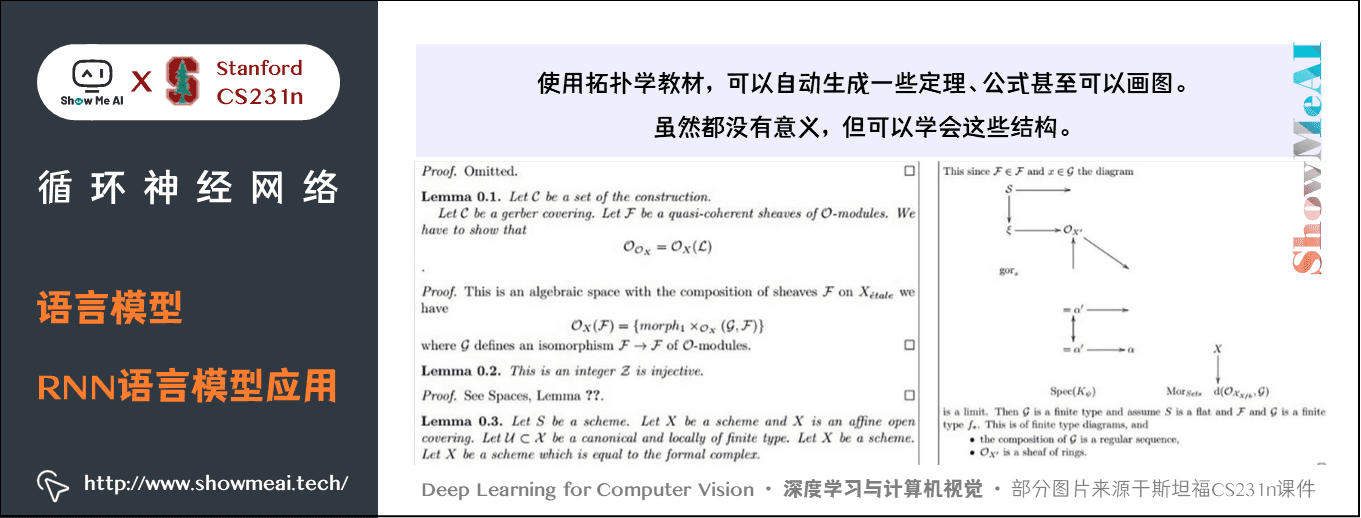• 使用 linux 源码进行训练。可以生成 C 代码，有缩进有变量声明甚至会写注释等等，看起来非常像 C 代码（当然代码逻辑不一定正常，编译会有很多错误）。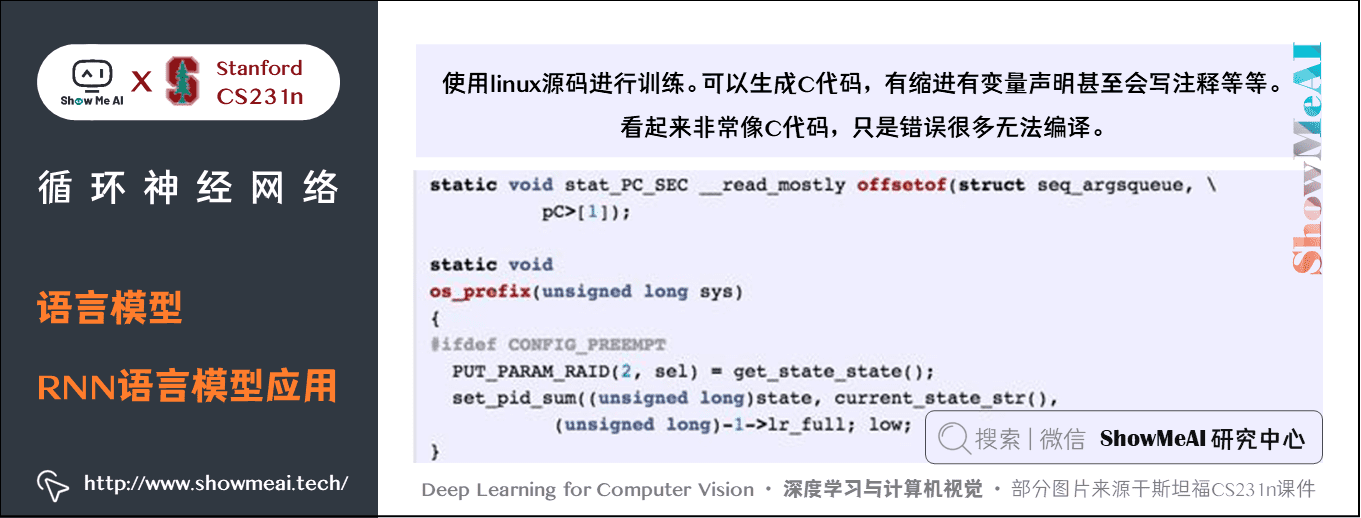### 2.3 RNN语言模型解释

• 蓝色位置数值低，红色位置数值高
• 比如某些元素遇到引号后，元素值会变得很低，然后一直保持很低直到下一个引号处被激活，元素值变大，然后保持到下一个引号再变低。所以有可能是检测引号的神经元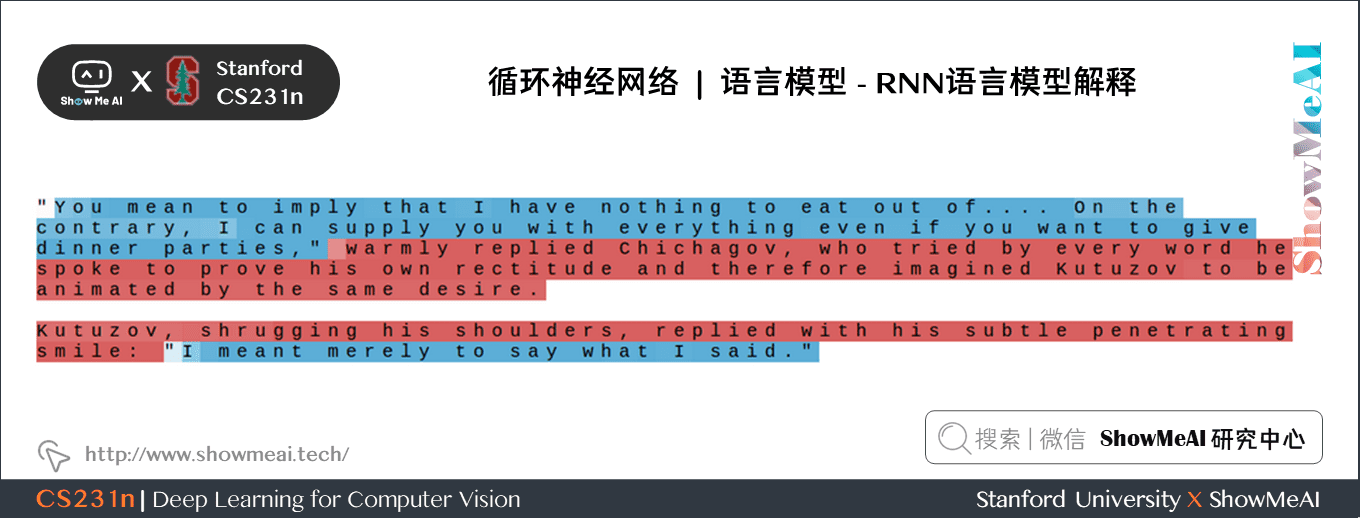• 还有某些神经元在统计回车符前的字符数量，即字符有多少时会自动换行。某一行开始处元素的值很低，然后慢慢增大，达到一定值后自动变成0，然后文本换行。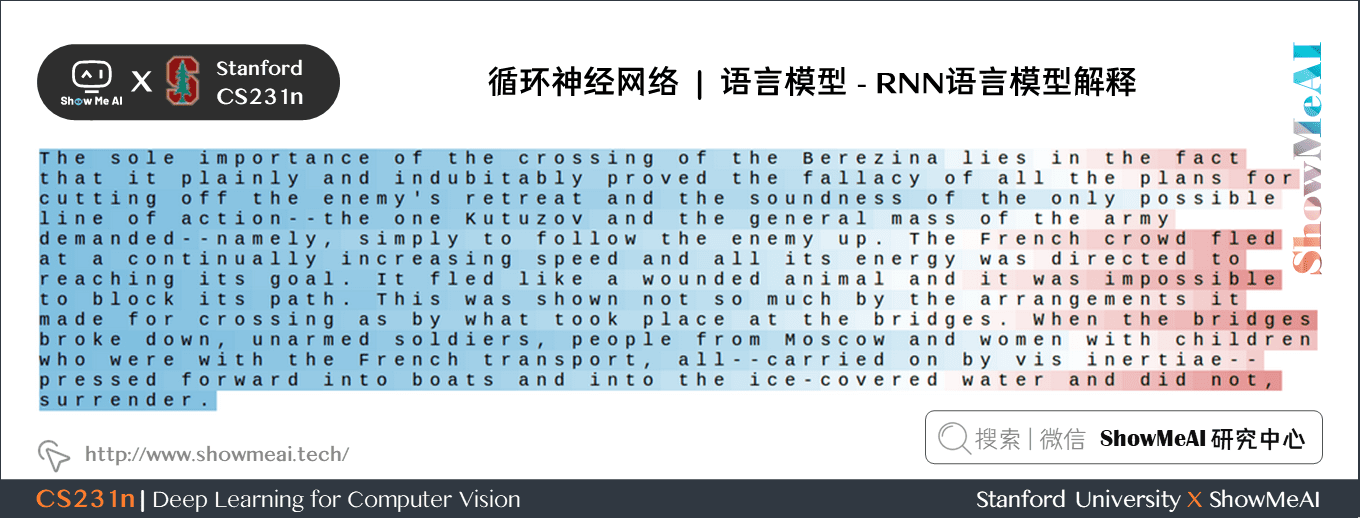• 在训练代码的例子中，有些神经元似乎在判断是否在
if
语句，是否在注释内，以及表示不同的缩进层级。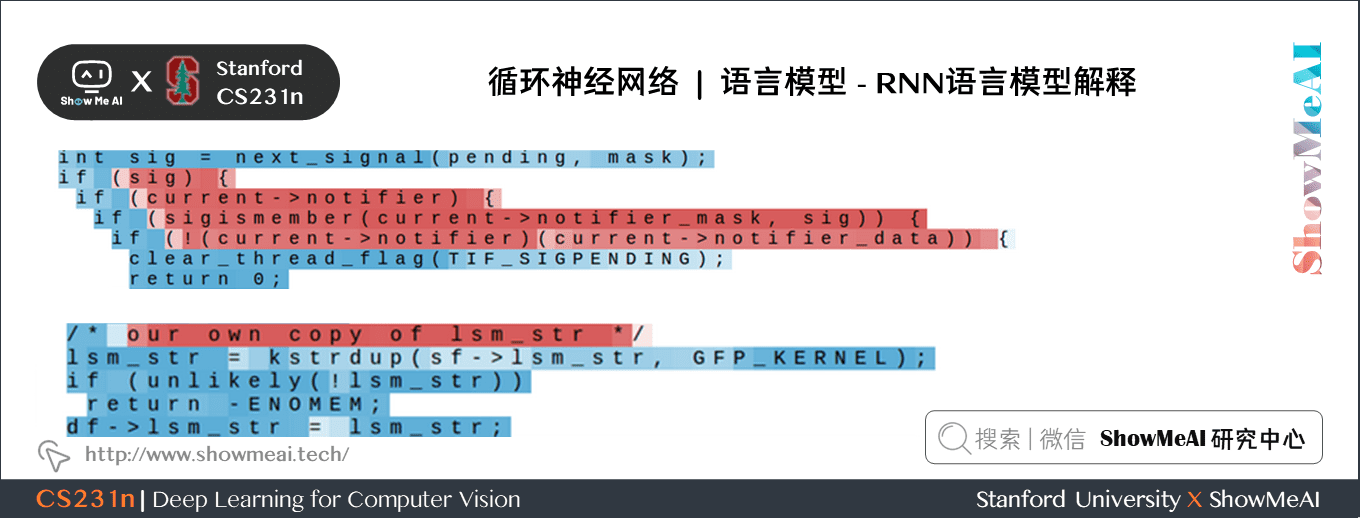## 3.看图说话、视觉问答、注意力模型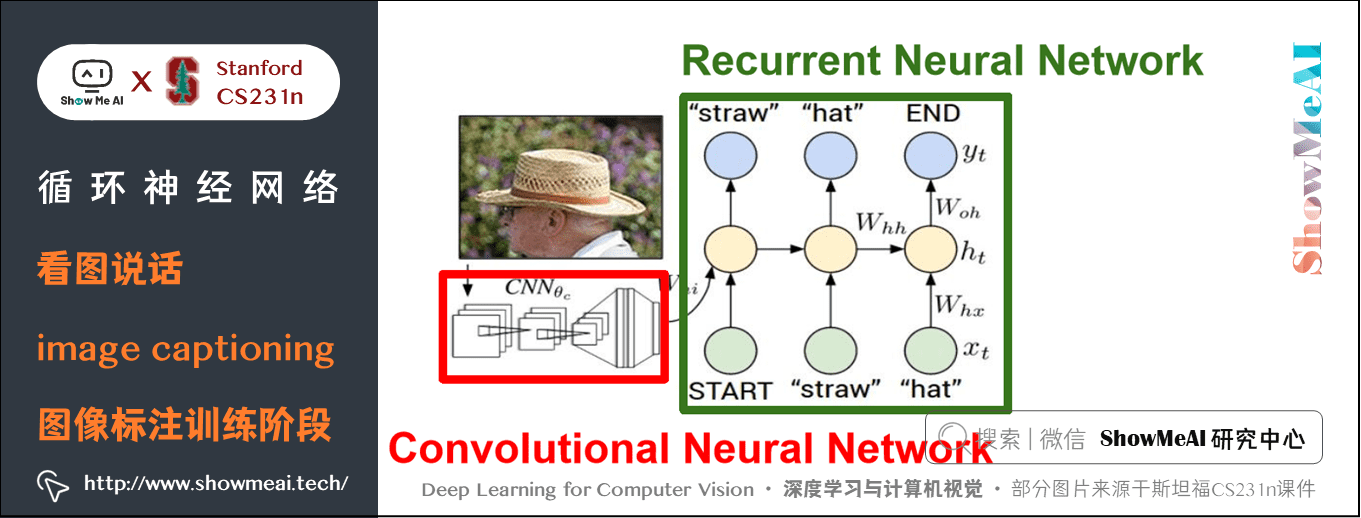• 我们把测试图像输入到卷积神经网络，通过 CNN 得到模型最后1个全连接层之前的1个图像向量，作为整张图像的内容表征。
• 之后会给语言模型输入一个开始标志，告诉模型开始生成以这个图像为条件的文本。不同于以往的隐状态公式，在这个任务中我们会把图像信息输入到每个时间步用于更新隐状态：
h = tanh(Wxh \ast x + Whh \ast h + Wih \ast v)
• 现在就可以根据图像的内容生成一个词汇表（有很多词汇）中所有单词的一个 softmax 得分概率分布，sample/取样之后作为下一个时间步的输入。
• 直到取样到「结束符号」整个预测过程结束，文本也生成完毕。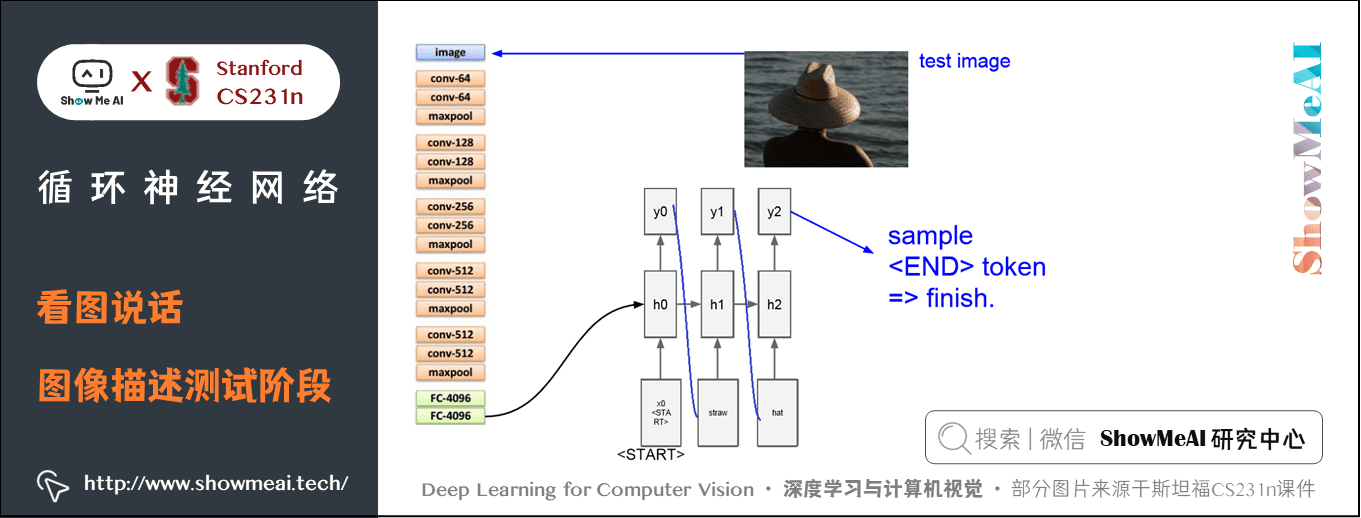### 3.1 基于注意力的「看图说话」模型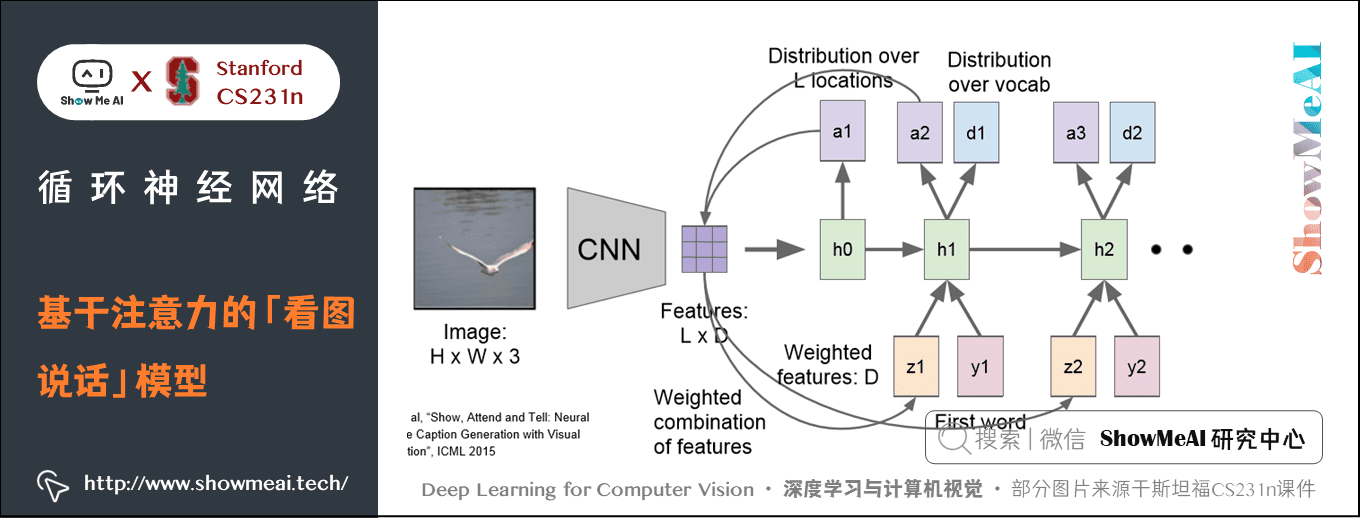z = \sum_{i=1}^L {p_iv_i}

p_i 就是基于位置的分布，v_i 就是 L 个特征向量中的一个，最后得到一个统计向量 $z$。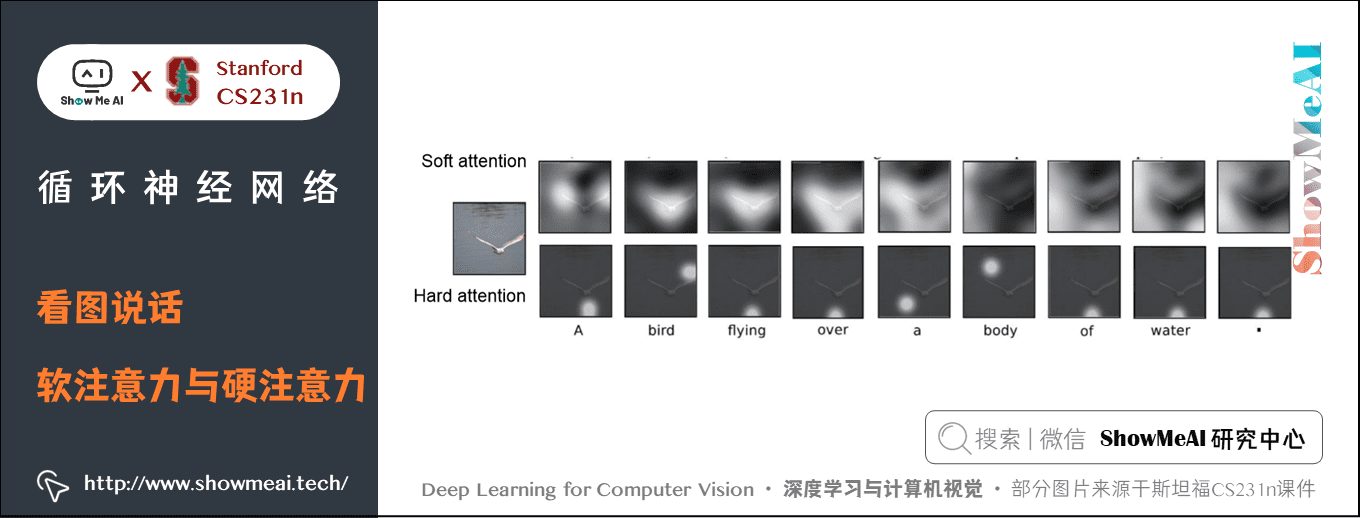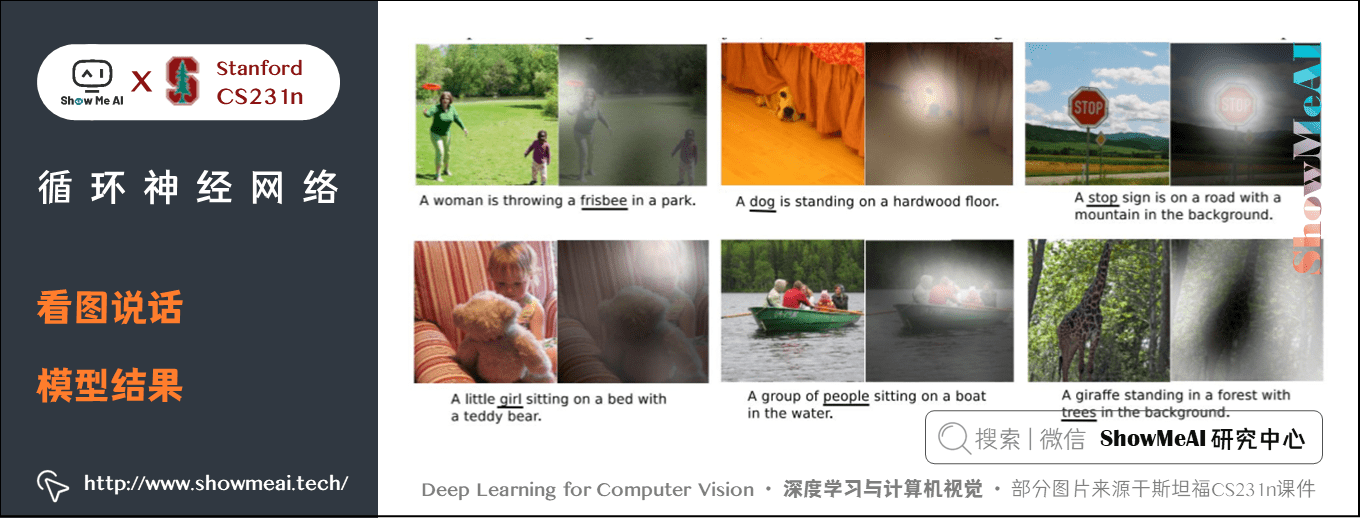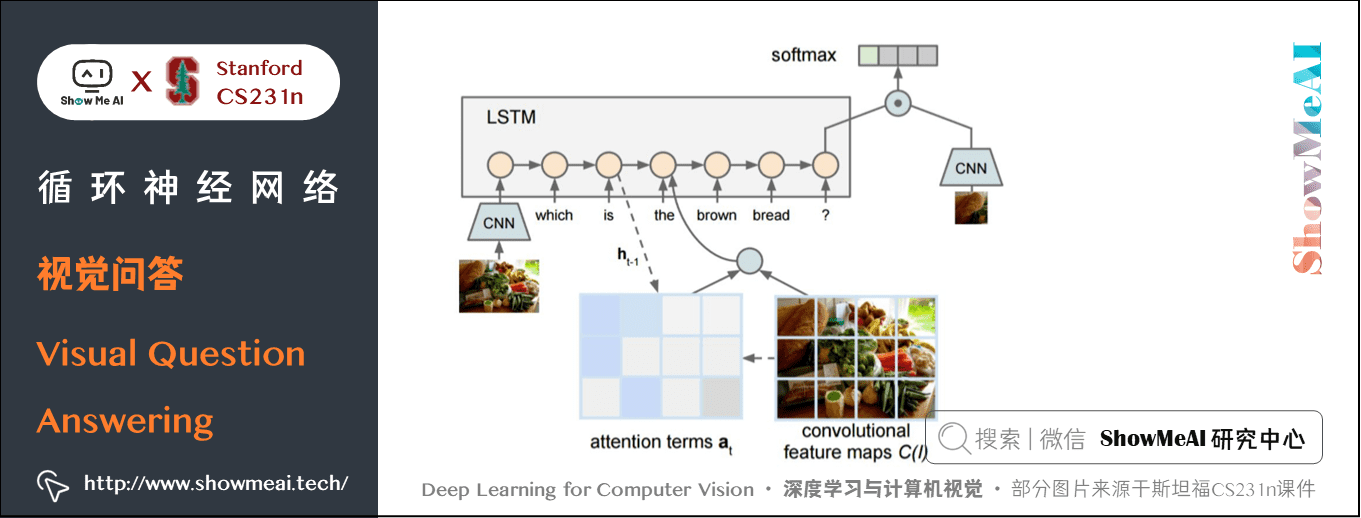## 4.RNN梯度流

### 4.1 多层RNN（Multilayer RNNs）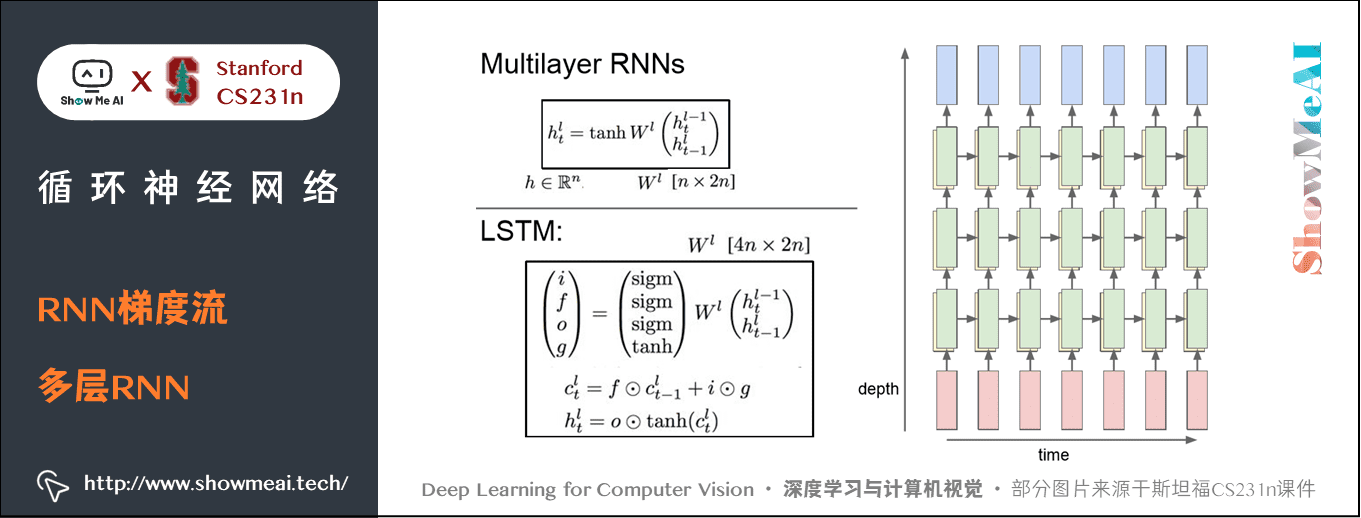### 4.2 普通RNN梯度流

\begin{aligned} h_t & = tanh(W_{hh}h_{t-1}+W_{xh}x_t) \\ & = tanh \bigl (\bigl (\begin{matrix}W_{hh} \\ &W_{xh} \end{matrix}\bigr)\bigl(\begin{matrix}h_{t-1}\\x_{t} \end{matrix}\bigr)\bigr) \\ & =tanh\bigl(W\bigl(\begin{matrix}h_{t-1}\\ x_{t} \end{matrix}\bigr)\bigr) \end{aligned}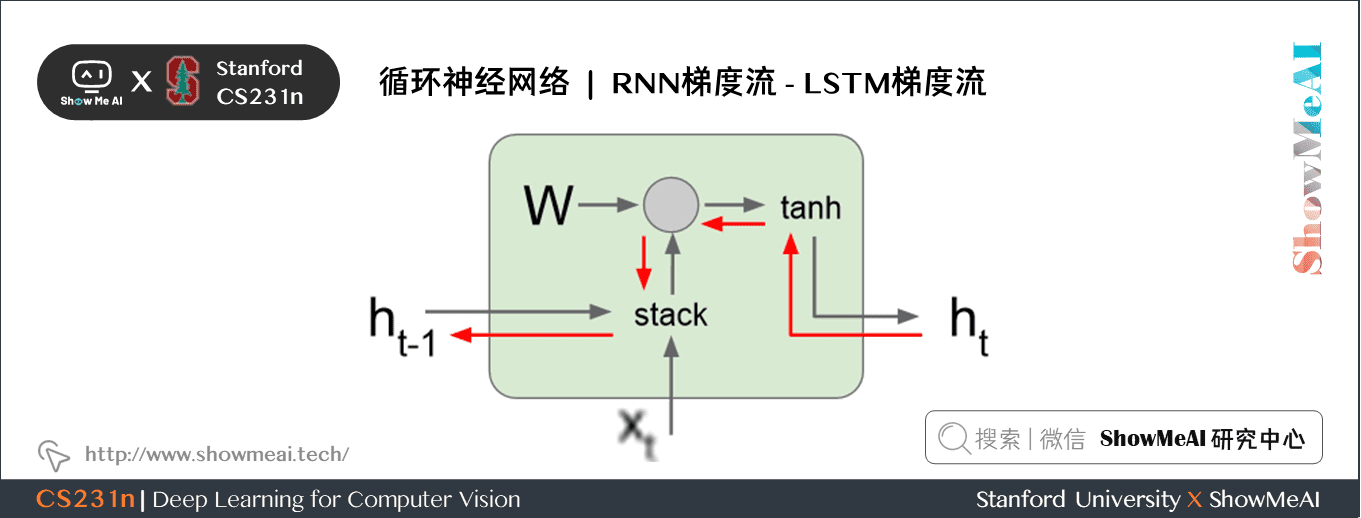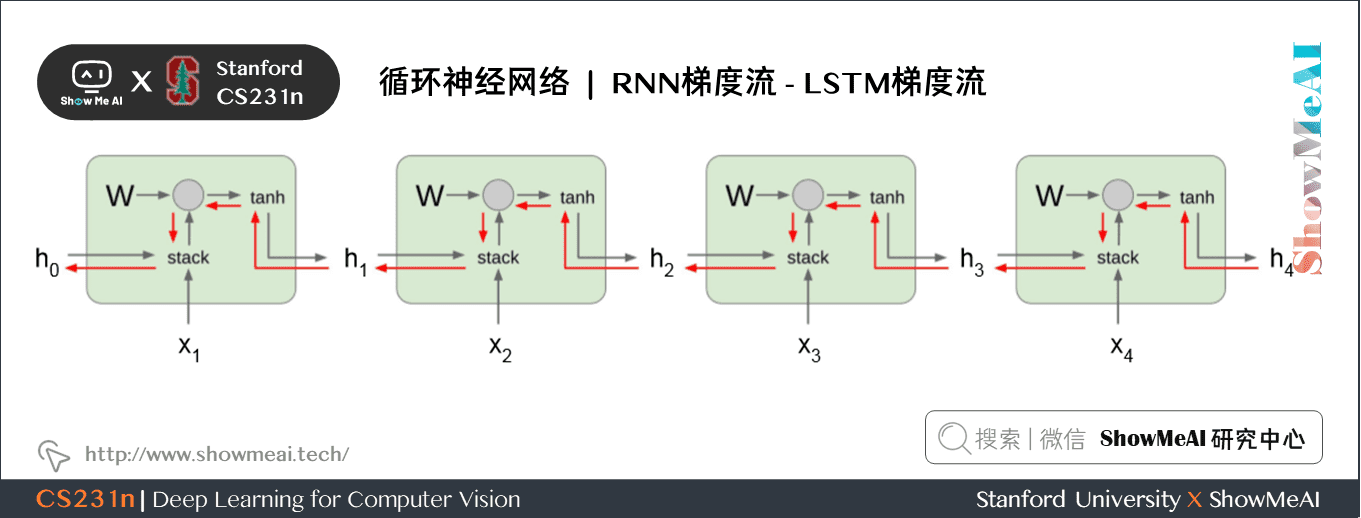grad_num = np.sum(grad * grad)
grad *= (threshold / grad_num)

### 4.3 LSTM（Long Short Term Memory ）

LSTM（长短期记忆）网络就是用来解决「梯度爆炸」和「梯度消失」问题的，与其在输出上限制梯度，LSTM 的网络结构更加复杂。

\begin{aligned} \left(\begin{array}{l} i \\ f \\ o \\ g \end{array}\right) &=\left(\begin{array}{c} \sigma \\ \sigma \\ \sigma \\ \tanh \end{array}\right) W\left(\begin{array}{c} h_{t-1} \\ x_{t} \end{array}\right) \\ c_{t} &=f \odot c_{t-1}+i \odot g \\ h_{t} &=o \odot \tanh \left(c_{t}\right) \end{aligned}

LSTM 在每一个时间步都会维持两个隐状态：

• h_t 和普通 RNN 中的一致，是 RNN 网络的隐状态；
• 单元状态向量 c_t ，是保留在 LSTM 内部的隐状态，不会完全暴露到外部去。计算公式可以看出，LSTM会使用输入和之前的隐状态来更新四个组成 c_t 的门，然后使用 c_t 来更新 h_t .

LSTM 中的权重矩阵计算会得到 4 个与隐状态 h 大小相同的向量，然后分别通过不同的非线性函数就得到了单元状态 $c_t$ 的四个门： i, f, o, g

• i 是输入门（Input gate） ，表示有多少内容被写到单元状态；
• f 是遗忘门（Forget gate），表示对之前的单元状态的遗忘程度；
• o 是输出门（Output gate） ，表示单元状态输出多少给隐状态；
• g 是门值门（Gate gate ） ，控制写入到单元状态的信息。

• f 是对之前的单元状态的遗忘，如果是 0 全部遗忘，如果是 1 就全部保留，那个圆圈加点的符号表示逐元素相乘；
• i 和 g 共同控制写入到单元状态向量的信息，i 在 0 \sim1 之间，g 在 -1 到 1 之间，这样每个时间步，单元状态向量的每个元素最大自增 1 或最小自减 1。
• 这样 c_t 可以看成是对 [-1 \quad 1] 的按时间步计数。然后 c_t 通过 tanh() 将这个计数压缩到 [0 \quad 1] 范围，然后 o 来控制将多少单元状态的信息输出给隐状态 h_t 。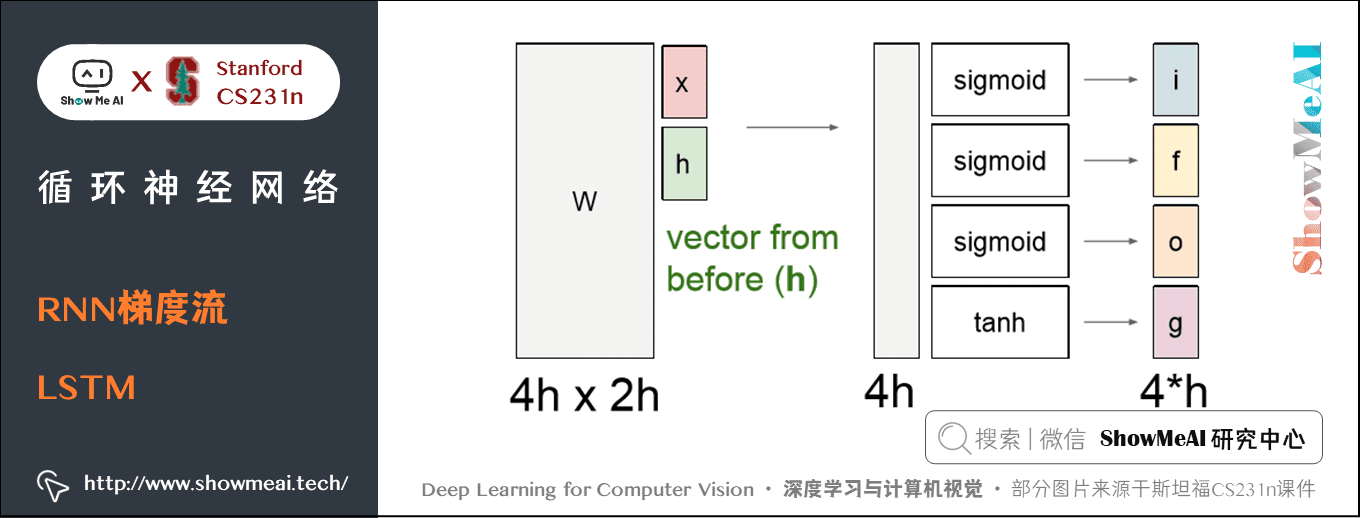LSTM梯度流

LSTM不同的时间步 f 的值都不同，不像普通 RNN 每次都乘相同的 W，这样就一定程度避免梯度爆炸或锐减。而且 f 的值也是在 [0, 1] 性质非常好。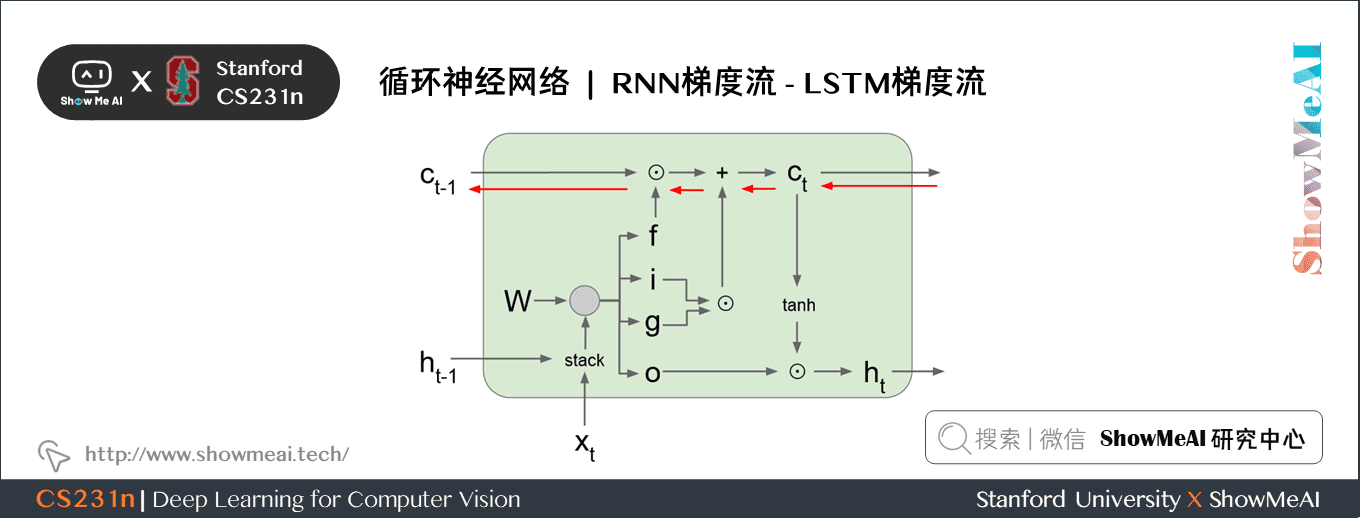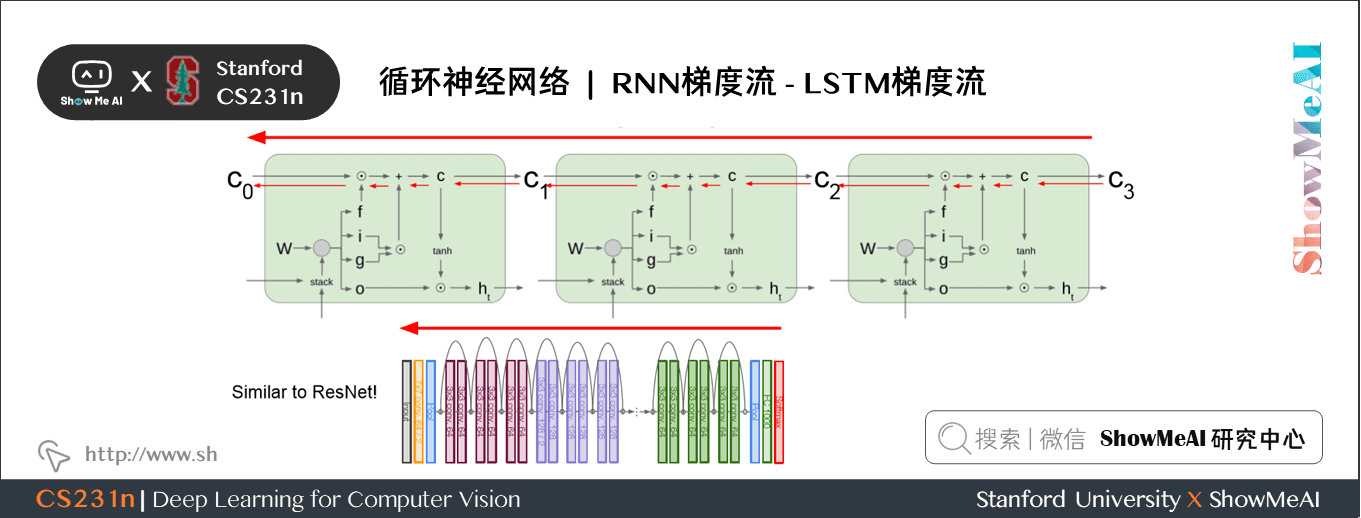### 4.4 GRU（Gated Recurrent Unit）

\begin{aligned} r_{t} &=\sigma\left(W_{x r} x_{t}+W_{h r} h_{t-1}+b_{r}\right) \\ z_{t} &=\sigma\left(W_{x z} x_{t}+W_{h z} h_{t-1}+b_{z}\right) \\ \tilde{h}_{t} &=\tanh \left(W_{x h} x_{t}+W_{h h}\left(r_{t} \odot h_{t-1}\right)+b_{h}\right) \\ h_{t} &=z_{t} \odot h_{t-1}+\left(1-z_{t}\right) \odot \tilde{h}_{t} \end{aligned}

## 7.要点总结

• RNN 是一种输入输出都很灵活的网络结构；
• Vanilla RNNs 很简单，但是效果不好；比较普遍的是使用 LSTM 和 GRU，能有效的改善梯度流；
• RNN 反向传播梯度爆炸可以给梯度设置阈值，而梯度锐减可以使用复杂的网络，比如 LSTM；
• RNN 的应用：图像标注、视觉问答，都是将 CNN 与 RNN 结合起来；
• 理解语言模型与注意力模型；

## ShowMeAI 系列教程推荐